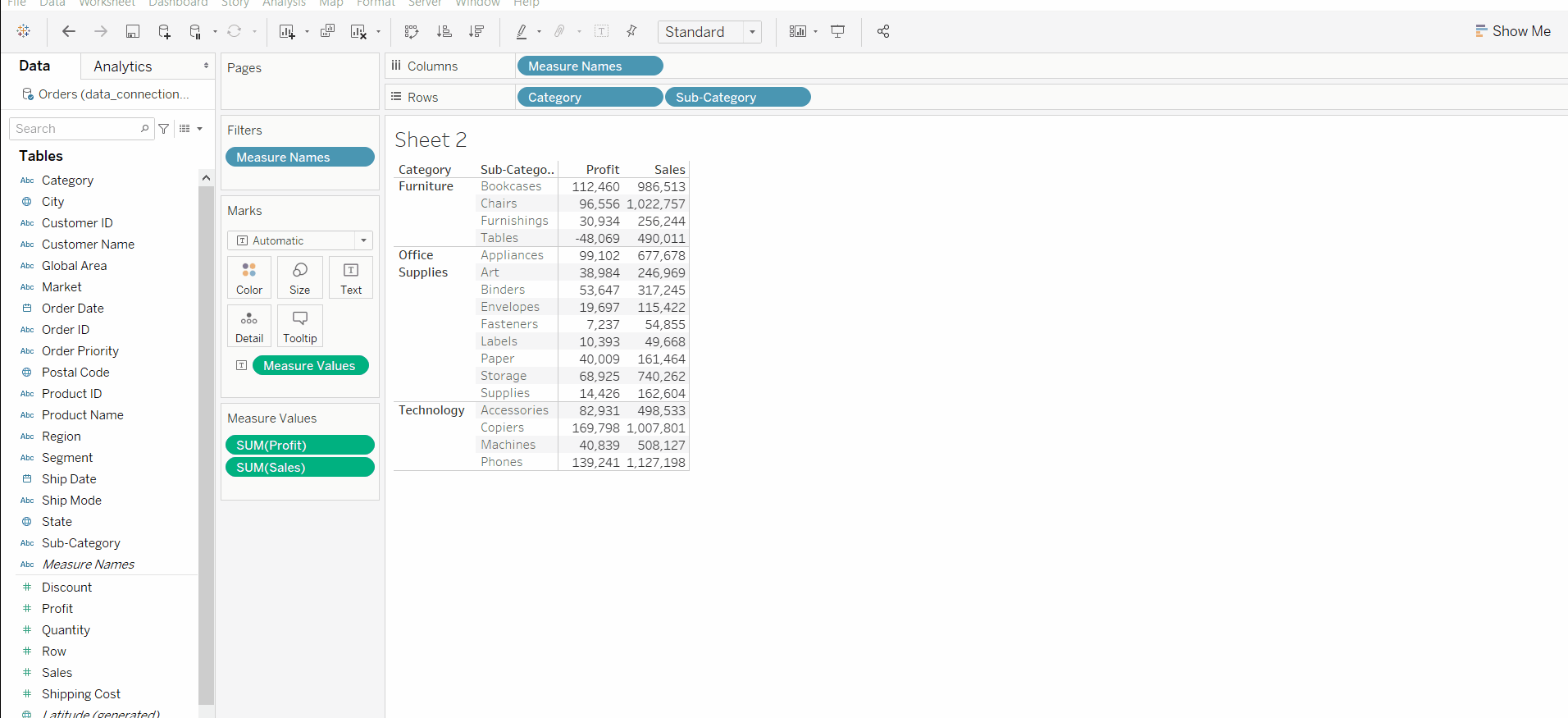Open In App
Related Articles
• Write an Interview Experience
• Tableau Tutorial

# Total in a crosstab by column in Tableau

In this article, we will learn how to calculate the total in a crosstab by column in tableau worksheet to do further operations. For this first look into two terms :

• Tableau: Tableau is a very powerful data visualization tool that can be used by data analysts, scientists, statisticians, etc. to visualize the data and get a clear opinion based on the data analysis. Tableau is very famous as it can take in data and produce the required data visualization output in a very short time.
• Crosstab: A crosstab may be defined as the data in form of a table with a cross(merging). In Tableau, the crosstab is the data representation with some attributes.

Dataset used in the given examples is Dataset.

For this we have to follow some steps :

Open the Tableau tool and connect a dataset into it. Drag and drop the one sheet of the connected dataset. Click on sheet1 to open the tableau worksheet. On clicking Sheet1 you will get whole dataset attributes on the left side and a worksheet for work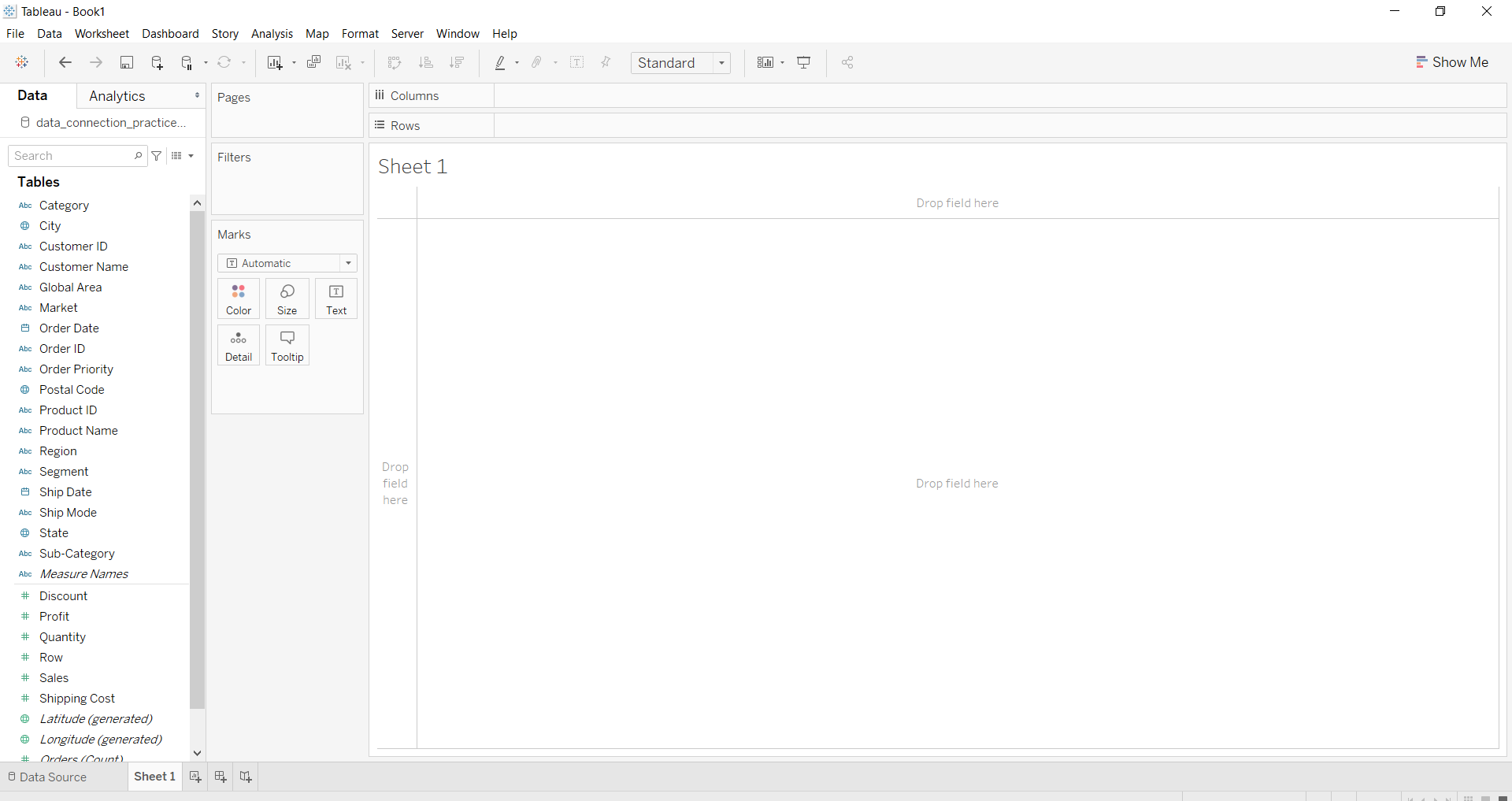To calculate total you have to cross tab by drag and drop ans select the chart option as crosstab.

Example 1: In this example, we add all subtotals.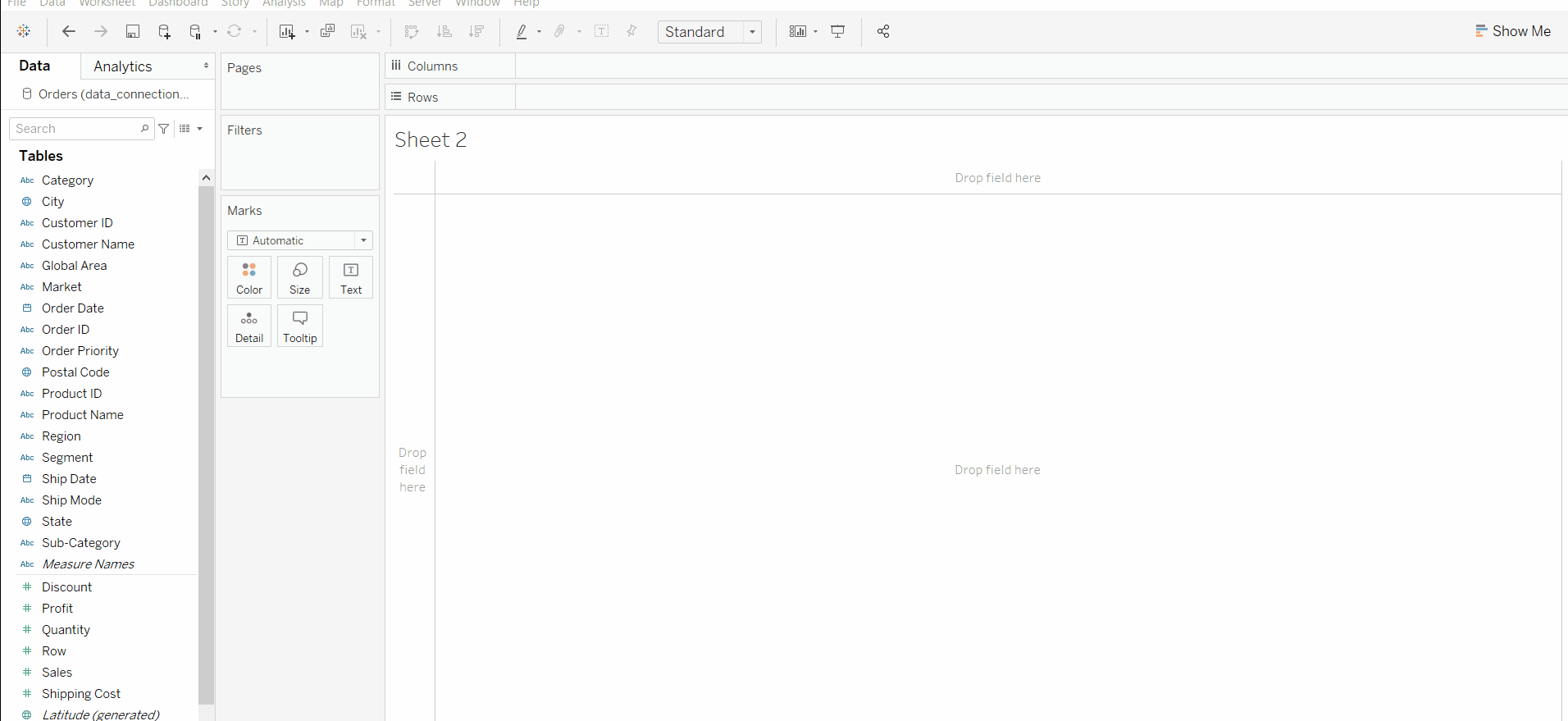Example 2: In this example, we calculated the grand total of columns.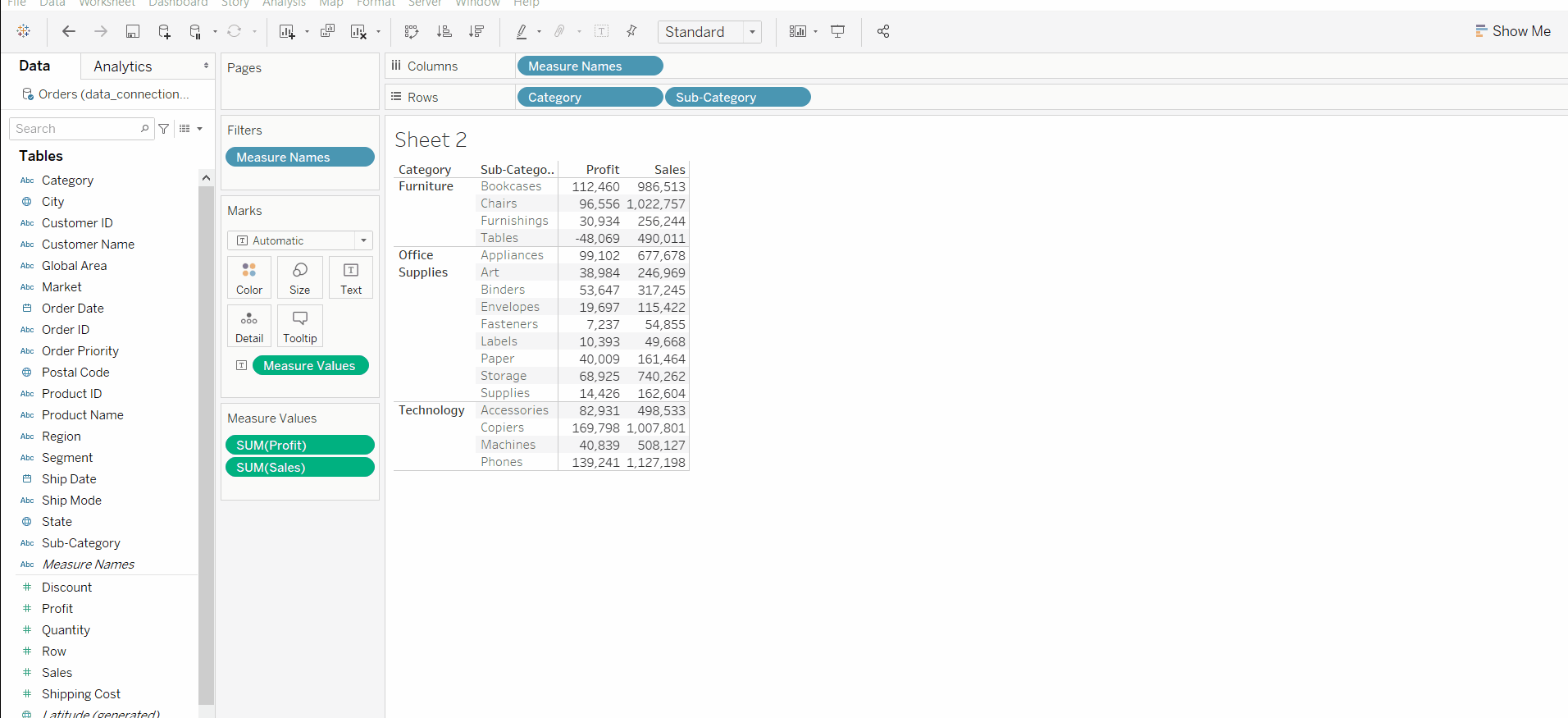Example 3: In this example we remove all subtotals.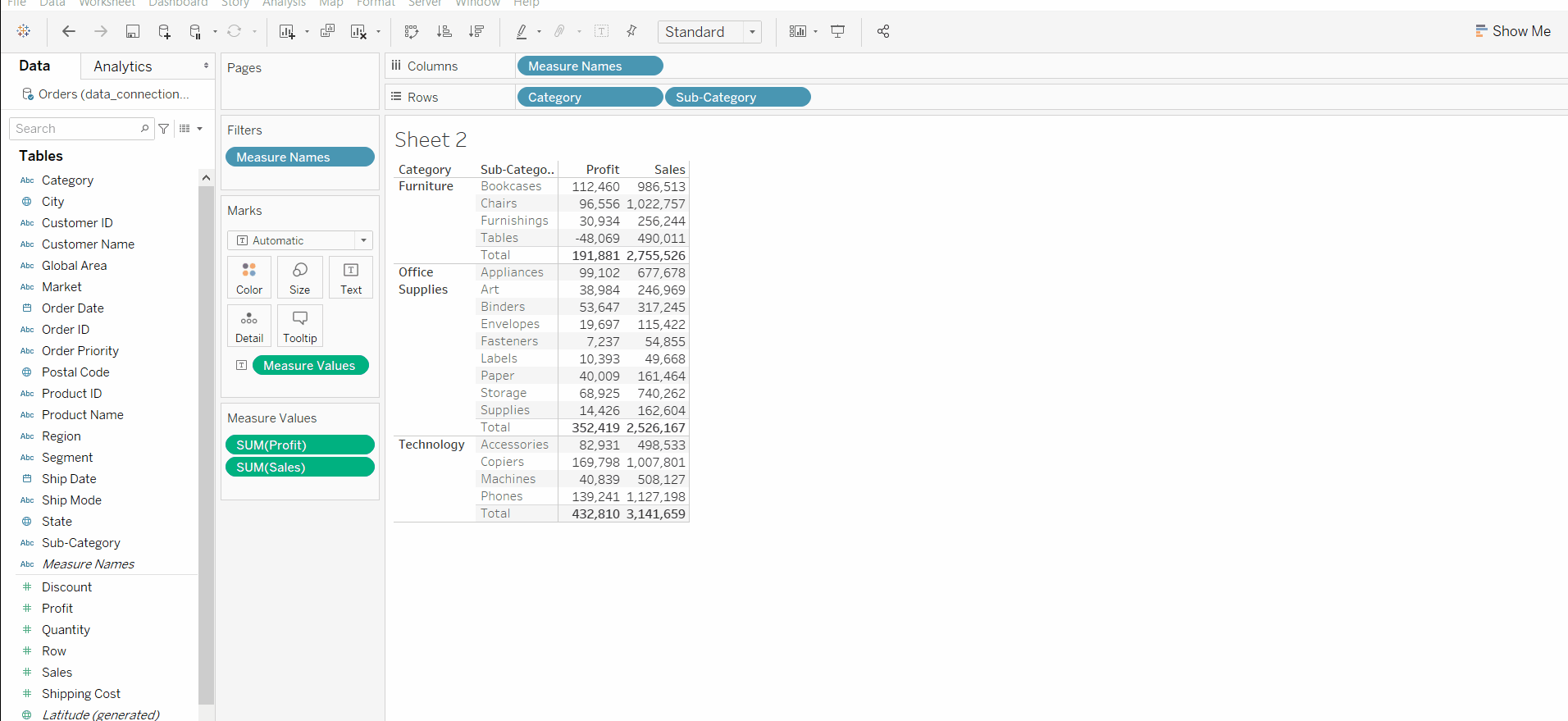Example 4: In this example, we add all subtotals and change the function used for the total.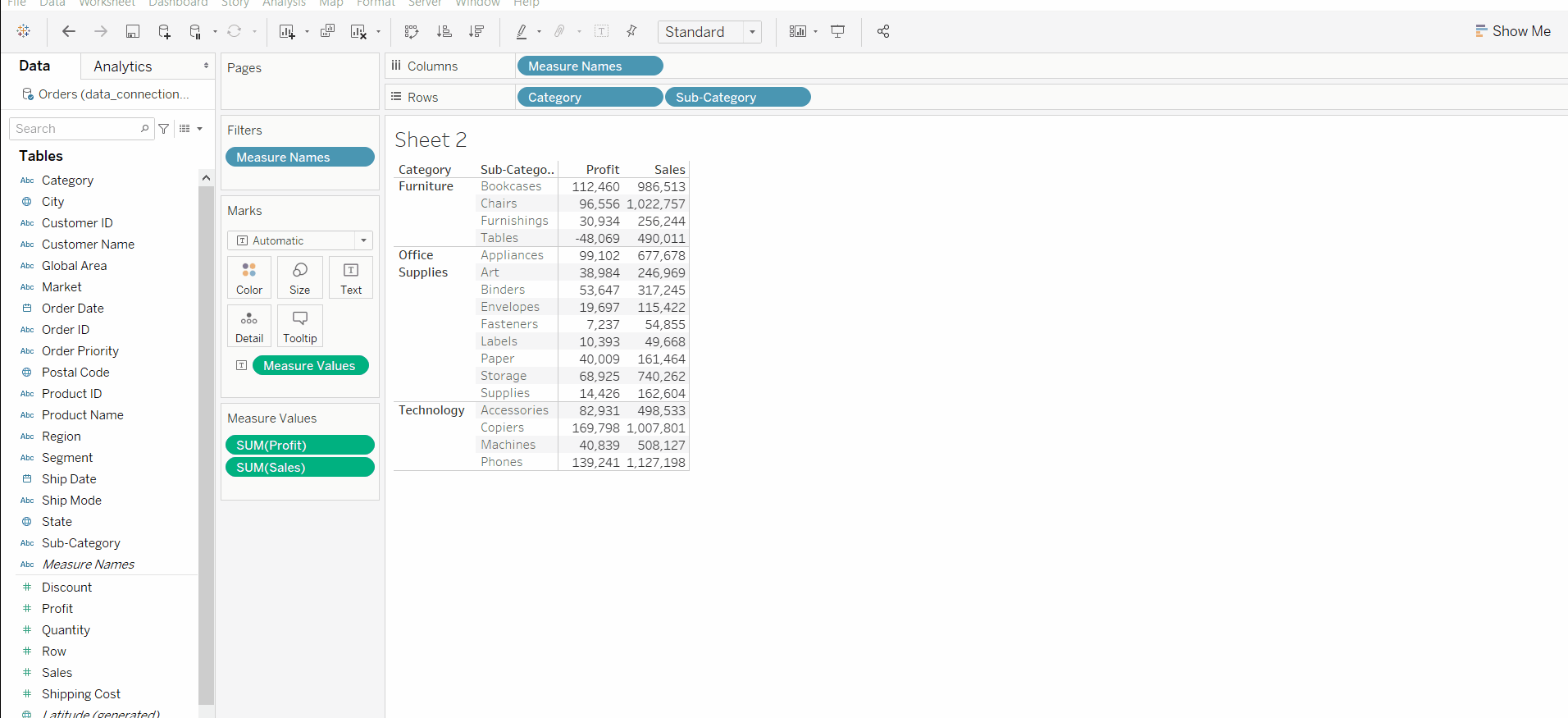Example 5: In this example, we add all subtotals then arrange the total at the top.